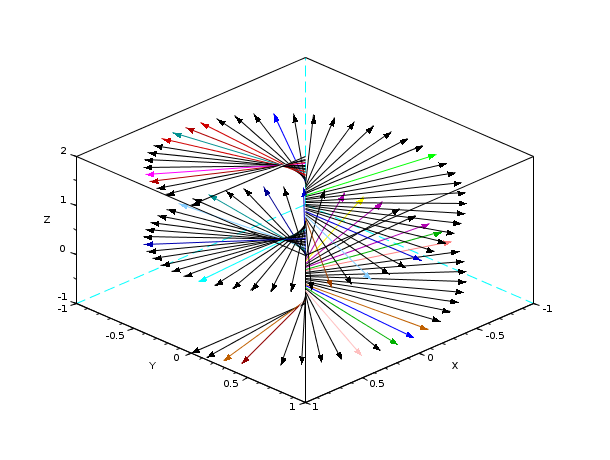Scilab Home page | Wiki | Bug tracker | Forge | Mailing list archives | ATOMS | File exchange
Change language to: Français - Português - 日本語 - Русский

See the recommended documentation of this function

Scilab Help >> Graphics > geometric_shapes > xarrows

# xarrows

draw a set of arrows in 2D or in 3D.

### Calling Sequence

`xarrows(nx, ny [,nz] [,arsize, color])`

### Arguments

nx, ny, nz

real vectors or matrices of same size.

arsize

a real scalar, size of the arrow head. The default value can be obtained by setting `arsize` to `-1`.

color

a matrix or scalar. If `color` is a positive scalar it gives the color to use for all arrows. If it is a negative scalar then the current color is used. If it is a vector `color(i)` gives the color to use for arrow `i`.

### Description

`xarrows` draws a set of arrows given by `nx`, `ny` and `nz`. If `nz` is not given, then the arrows are drawn in 2D. If `nx`, `ny` and `nz` are vectors, the `i`-th arrow is defined by `(nx(i),ny(i),nz(i))-->(nx(i+1),ny(i+1),nz(i+1))`. If `nx`, `ny` and `nz` are matrices:

```nx=[xi_1 x1_2 ...; xf_1 xf_2 ...]
ny=[yi_1 y1_2 ...; yf_1 yf_2 ...]
nz=[zi_1 z1_2 ...; zf_1 zf_2 ...]```

the `k`-th arrow is defined by `(xi_k,yi_k,zi_k)-->(xf_k,yf_k,zf_k)`.

`xarrows` uses the current graphics scale which can be set by calling a high level drawing function such as `plot2d`.

### Examples

```x = 2*%pi*(0:7)/8
x1 = [sin(x); 9*sin(x)];
y1 = [cos(x); 9*cos(x)];
plot2d([-10,10], [-10,10], [-1,-1], "022")
xset("clipgrf")
xarrows(x1, y1, -1, [1:7,9]) //8-th color is white
xset("clipoff")``````N = 100;
zi = linspace(-1,2,N);
zf = zi;
theta = (zi - min(zi))/(max(zi)-min(zi))*720;
xi = zeros(1,N);  xf = cosd(theta);
yi = zeros(1,N);  yf = sind(theta);
clf, plot3d([-1 1 1 1],[-1 -1 -1 1],[-1 -1 2 2])
xarrows([xi;xf],[yi;yf],[zi;zf],0.7, 32*rand(1,N))```### History

 Version Description 5.5.0 Arrows can be drawn in 3D.